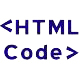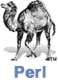## HTML For the Quiz

<form action="http://jacknilan.tripod.com/cgi-bin/quiz.pl" method="post" >
1. Who was first president of the US? <br>
<input name="Q1" value="1" TYPE="radio" > a. Lincoln
<br> <input name="Q1" value="2" TYPE="radio" > b. Jefferson <br>
<input name="Q1" value="3" TYPE="radio" > c. Washington <br>
<input name="Q1" value="4" TYPE="radio" > d. Adams <br>
<input name="Q1" value="5" TYPE="radio" > e. Monroe <br>
<br>
2. Who was the second President of the US? < br >
<input name="Q2" value="1" TYPE="radio" > a. Lincoln <br>
<input name="Q2" value="2" TYPE="radio" > b. Washington <br>
<input name="Q2" value="3" TYPE="radio" > c. Jefferson <br>
<input name="Q2" value="4" TYPE="radio" > d. Adams <br>
<input name="Q2" value="5" TYPE="radio" > e. Monroe <br>
<input name="Q2" value="6" TYPE="radio" > f. Clinton <br>

< INPUT TYPE="SUBMIT" name="prespage" value=" Score " > < br>
< /form>## Perl Script to Correct Quiz

#!/usr/local/bin/perl
use CGI qw(param);
\$a = param('Q1');
\$b = param('Q2');
\$ct = 0;
print "Content-type: text/html\n\n";
print "<html>";
print "<body>";
print "<h2>President's Quiz Results </h2>";
if (\$a eq "3")
{\$ct++;}
else
{print "Correct answer for #1 was 3<br><br>"; }
if (\$b eq "4")
{\$ct++;}
else
{print "Correct answer for #2 was 4<br><br>"; }
print "Number right was \$ct \n\n";
print "Score was".\$ct/2.0*100;
print "</body></html>\n";